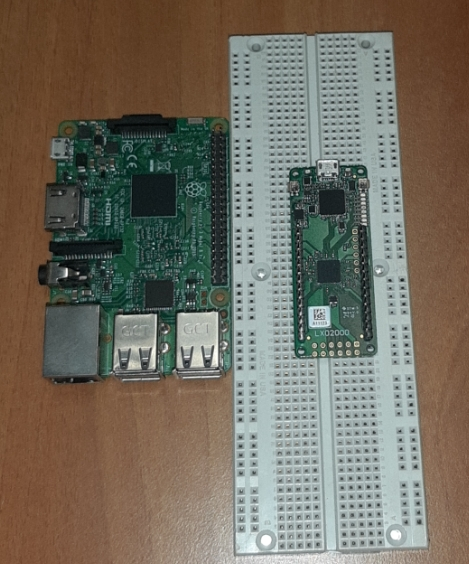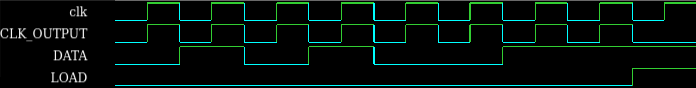# Can the Raspberry pi acquire high frequency signals?

We bench marked two of the most popular python libraries. The best one managed to acquire signals with accuracy above 99% for frequencies up to 20 KHz. Above that frequency, its performance gradually deteriorates and at 110 KHz it cannot perform the task. Let’ take a closer look at the experiment.

## Introduction#

The Raspberry Pi (RPi) is undoubtedly a revolutionary affordable single board computer, with multiple applications and a must have accessory for every hardware hacker and maker. Gradually though, it is increasingly getting traction and is being used on main stream commercial applications.

We have experimented with it a lot and used it in a few of our IoT projects and the compelling argument for its use is that it is rather easy to develop on it more complex IoT applications, that require the deployment of services such us a web server, a complex GUI running on a connected monitor or connection to external web servers.

On the other hand, its great strength is at the same time its greatest weakness, when it comes to signal acquisition. As the RPi is a single board computer running a fully fledged operating system, it has to dedicate resources to OS processes, while at the same time reading the input signals, something that could have a negative effect when working with high frequency inputs. Hence a key question arises.

“What are the Raspberry Pi’s capabilities with regard to high frequency synchronous signal acquisition?”

We have put the RPi to the test and benchmarked two of the most popular python libraries, RPi.GPIO and PIGPIO, with regard to their performance in accurately reading interdependent high frequency input signals (i.e 1 MHz to 5MHz). In this post we are sharing our findings.

## Scope of benchmark testing#

One of the fundamental electronic components is the serial-in, parallel-out (SIPO) shift register. In (SIPO) shift registers, the data is stored into the register serially, while it is retrieved from it in parallel fashion. At each clock tick the data within the register moves to a higher order position by a single bit, while a new bit of the input phrase enters into the register.

In a slightly more complected design, a D Flip-Flop, synchronized separately, can be added to the parallel output of the SIPO shift register in order to store the data.

In this experiment, we converted the Raspberry Pi into an 8-bit SIPO shift register, connected to an 8 bit D Flip-Flop. The aim was to feed the raspberry with a known 8-bit “phrase” and measure the amount of times per minute this phrase was read accurately, for different frequencies.

### Equipment & Software#

For this test we used a Raspberry Pi 3B running Raspberry Pi OS Lite (Kernel version: 5.4). In order to generate the signals we used a Lattice MachXO2-4000 FPGA, integrated on a third party development board equipped with a 100 MHz MEMS oscillator.In case you are interested in replicating the experiment, lets go through every step of the set up required for this test. The required code for this test is available on github.

### Step 1 – Creating the input signals#

The following single bit input signals are required:

• DATA, carrying the serial data
• CLK, the clock. On the falling edge of CLK the value of Data is loaded into the SIPO shift register
• a LOAD signal. On the falling edge of LOAD, the parallel output of the SIPO shift register is to be read and compared to the known phrase “01010011”

The timing diagram for the above signals is shown in the following image:In the following VHDL component can be used in order to program the above behavior into the FPGA:

----
library ieee;
use ieee.std_logic_1164.all;
use ieee.std_logic_unsigned.all;
entity counter_input is
port(
clk: in std_logic;
);
end counter_input;
architecture behavioral of counter_input is
begin
process (clk)
variable count: integer:= 1;
begin
if falling_edge(clk) then
if count=1 then
DATA <= '0';
elsif count=3 or count=5 or count=6 then
DATA <= '0';
elsif count=2 or count=4 or count=7 or count=8 then
DATA <= '1';
elsif count=9 then
count:=0;
end if;
count:=count+1;
end if;
end process;
begin
CLK_OUTPUT <= clk and NOT temp_LOAD;
end process;
end behavioral;

In addition, as the onboard oscillator runs at 100 MHz, a frequency divider was used, creating the required frequencies for the experiment. You can find the complete VHDL script for programming the FPGA here.

##### tip

The FPGA is programmed to transition the signals on the falling edge of the clock

### Step 2 – Programming the Raspberry Pi#

To preserve system resources we used the Raspberry Pi OS Lite. On a fresh image, we installed only the minimum required packages.

In order to accurately measure the read accuracy of the Raspberry Pi's GPIO pins at different frequencies, we wrote a simple script, for each of the tested python libraries, that uses three of GPIO pins as inputs, each corresponding to the DATA, CLK and LOAD signals. The script samples the DATA pin on the rising edge of the CLK signal and appends this value to the input phrase. On te rising edge of the LOAD signal, it compares the received phrase with the expected one (“01010011”).

This process is executed for 60 seconds and at the end of this period it calculates the read accuracy for the respective frequency as:

accuracy = correct count / total count

The calculation is repeated for different input frequencies in order to get an Accuracy - Frequency curve.

##### tip

The RPi is programmed to detect rising edge transitions of the two control signals (CLK and LOAD) in contrast with the FPGA

Our code for testing the RPi.GPIO library is as follows:

import RPi.GPIO as GPIO
import time
def onRising(gpio):
global DATA_LEVEL, phrase, count, correct_count, init
if gpio == CLK_pin:
phrase += str(DATA_LEVEL)
if not init:
init = True
else:
count += 1
if phrase == '01010011':
correct_count += 1
phrase=''
def onBoth(gpio):
global DATA_LEVEL
DATA_LEVEL=GPIO.input(gpio)
if __name__ == '__main__':
CLK_pin=17
DATA_pin=22
DATA_LEVEL=1
phrase=''
init = False
count = 0
correct_count = 0
GPIO.setmode(GPIO.BCM)
GPIO.setup(CLK_pin, GPIO.IN)
GPIO.setup(DATA_pin, GPIO.IN)
print("Starting benchmark")
time.sleep(60)
print('Frequency XX:',count,'-',correct_count)

Our code for testing the PIGPIO library is as follows:

import pigpio, time
def onChange(gpio,level,tick):
global data_level
global phrase, init
global count, correct_count
if gpio == DATA_pin:
data_level = level
if not init:
init = True
else:
count += 1
if phrase == "01010011":
correct_count += 1
phrase = ''
else: # clock pin
phrase = phrase + str(data_level)
if __name__ == '__main__':
DATA_pin=22
CLK_pin=17
phrase=""
data_level = 0
count = 0
correct_count = 0
init = False
pi = pigpio.pi()
pi.set_mode(CLK_pin, pigpio.INPUT)
pi.set_mode(DATA_pin, pigpio.INPUT)
cb1 = pi.callback(DATA_pin, pigpio.EITHER_EDGE, onChange)
cb2 = pi.callback(CLK_pin, pigpio.RISING_EDGE, onChange)
print('Start Benchmark')
time.sleep(60)
print('Frequency XX:',count,'-',correct_count)

### Step 3 – Connecting#

Before powering up the system we connected the three input signals, pin by pin and also wired a common ground to both of the boards.

## Results#

Both libraries perform well for frequencies of up to 5 KHz with an accuracy above 99%. The accuracy of the Rpi.GPIO library deteriorates over 5 KHz and at 50 KHz it is incapable of performing this task.

The PIGPIO library performs comparably better, with its accuracy being above 99% for frequencies up to 20 KHz. Above that frequency, its performance gradually deteriorates and at 110 KHz it cannot read correctly any phrase at all.

The complete results of the benchmark test are presented on the following chart.## Conclusion#

The Raspberry Pi 3B performs well as a device for data acquisition at low frequencies and can be a rather good choice. On the contrary, for tasks involving high frequency signal acquisition it is out performed.

We are planning to benchmark more communication protocols (SPI, I2C), libraries and devices, including the Raspberry Pi 4. If you are interested in this topic, you can subscribe to our mailing list and receive notifications about new posts, when we publish them.

If you have a suggestion for a new topic, please drop us an email at team@k-ren.gr